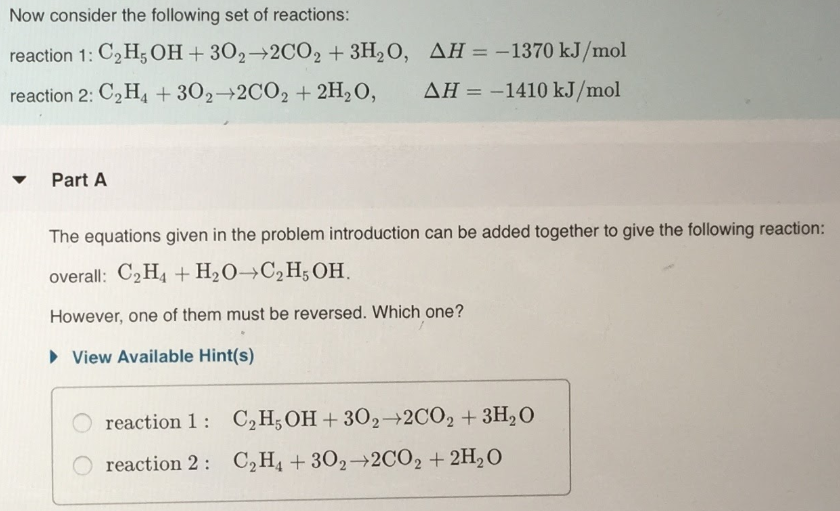# Now consider the following set of reactions: rection 1: C2H5OH + 3O2 → 2CO2 + 3H2O, ΔH = -1370kJ/mol reaction 2: C2H4 + 3O2 → 2CO2 + 2H2O, ΔH =1410 kJ/mol The equation given in the problem introduction can be added together to give the following reaction: overall: C2H4 + H2O → C2H5OH However, one of them must be reversed. Which one?